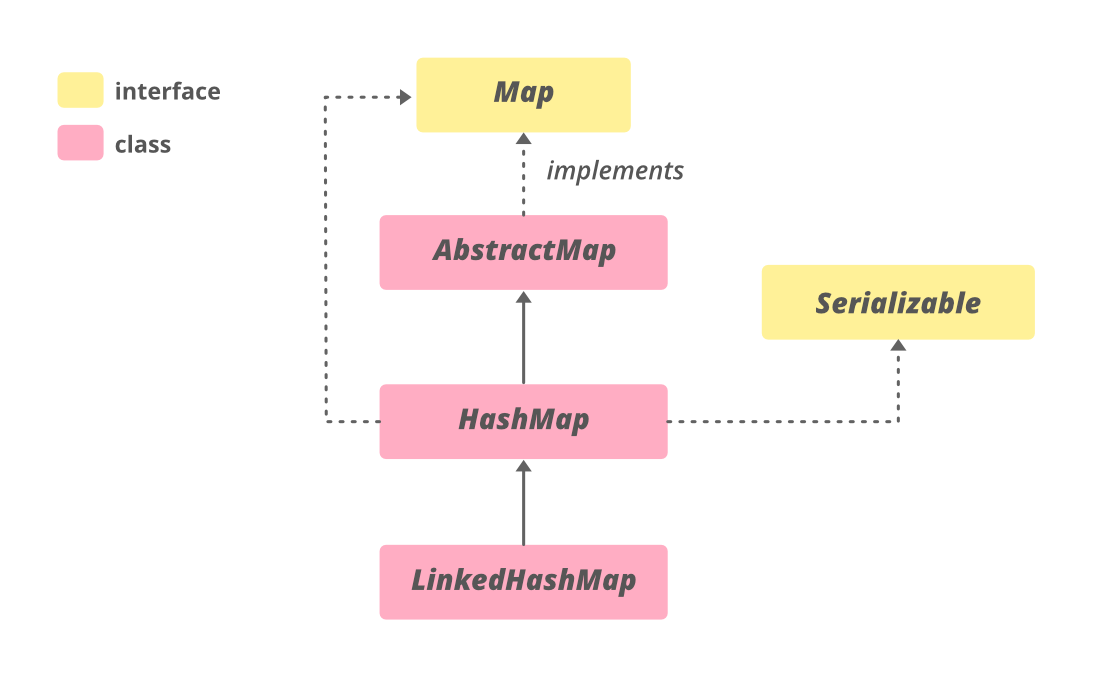Open In App

# Traverse Through a HashMap in Java

HashMap stores the data in (Key, Value) pairs, and you can access them by an index of another type. HashMap class implements Map interface which allows us to store key. hashMap is a part of the java collections framework been up since Java 1.2. It internally uses hashing technique which is pretty fast.Syntax:

```public class HashMap<K,V>
extends AbstractMap<K,V>
implements Map<K,V>, Clonnable, Serial     ```

### Different Ways of Traversal

We can iterate over mapping that is key and value pairs a was listed below that are later described as follows:

Methods:

1. Using an Iterator
2. Using enhanced for Loop (for-each loop)
3. Using forEach() Method

Method 1: Using an Iterator

Iterator is an interface in java.util package which is used to iterate through a collection. As such there is nothing special to discuss iterators so do we will be proposing out methods of Iterator interface been used to traverse over HashMap.

• hm.entrySet() is used to retrieve all the key-value pairs called Map.Entries and stores internally into a set.
• hm.entrySet().iterator() returns an iterator that acts as a cursor and points at the first element of the set and moves on till the end.
• hmIterator.hasNext() checks for the next element in the set and returns a boolean
• hmIterator.next() returns the next element(Map.Entry) from the set.
• mapElement.getKey() returns the key of the associated Map.Entry
• mapElement.getValue() return the value of the associated Map.Entry

Example:

## Java

 `// Java Program to Traverse through HashMap``// Using Iterator` `// Importing required classes``import` `java.util.*;` `// Main class``class` `GFG {` `    ``// Main driver method``    ``public` `static` `void` `main(String[] args)``    ``{` `        ``// Creating an Hashmap of string-integer pairs``        ``// It contains student name and their marks``        ``HashMap hm``            ``= ``new` `HashMap();` `        ``// Adding mappings to above HashMap``        ``// using put() method``        ``hm.put(``"GeeksforGeeks"``, ``54``);``        ``hm.put(``"A computer portal"``, ``80``);``        ``hm.put(``"For geeks"``, ``82``);` `        ``// Printing all elements of HashMap``        ``System.out.println(``"Created hashmap is"` `+ hm);` `        ``// Getting an iterator``        ``Iterator hmIterator = hm.entrySet().iterator();` `        ``// Display message only``        ``System.out.println(``            ``"HashMap after adding bonus marks:"``);` `        ``// Iterating through Hashmap and``        ``// adding some bonus marks for every student``        ``while` `(hmIterator.hasNext()) {` `            ``Map.Entry mapElement``                ``= (Map.Entry)hmIterator.next();``            ``int` `marks = ((``int``)mapElement.getValue() + ``10``);` `            ``// Printing mark corresponding to string entries``            ``System.out.println(mapElement.getKey() + ``" : "``                               ``+ marks);``        ``}``    ``}``}`

Output

```Created hashmap is{GeeksforGeeks=54, A computer portal=80, For geeks=82}
GeeksforGeeks : 64
A computer portal : 90
For geeks : 92```

Method 2: Using for-each Loop

Example:

## Java

 `// Java program for Traversing through HashMap``// Using for-each Loop` `// Importing required classes``import` `java.util.*;` `// Main class``class` `GFG {` `    ``// Main driver method``    ``public` `static` `void` `main(String[] args)``    ``{` `        ``// creating an empty HashMap of string and integer``        ``// pairs Mappings denotes Student name and marks``        ``HashMap hm``            ``= ``new` `HashMap();` `        ``// Adding mappings to HashMap``        ``// using put() method``        ``hm.put(``"GeeksforGeeks"``, ``54``);``        ``hm.put(``"A computer portal"``, ``80``);``        ``hm.put(``"For geeks"``, ``82``);` `        ``// Printing all elements of above Map``        ``System.out.println(``"Created hashmap is"` `+ hm);` `        ``// Display message only``        ``System.out.println(``            ``"HashMap after adding bonus marks:"``);` `        ``// Looping through the HashMap``        ``// Using for-each loop``        ``for` `(Map.Entry mapElement : hm.entrySet()) {``            ``String key = mapElement.getKey();` `            ``// Adding some bonus marks to all the students``            ``int` `value = (mapElement.getValue() + ``10``);` `            ``// Printing above marks corresponding to``            ``// students names``            ``System.out.println(key + ``" : "` `+ value);``        ``}``    ``}``}`

Output:

```Created hashmap is{GeeksforGeeks=54, A computer portal=80, For geeks=82}
GeeksforGeeks : 64
A computer portal : 90
For geeks : 92```

Method 3: Using forEach() method

forEach() is a method of HashMap that is introduced in java 8. It is used to iterate through the hashmap and also reduces the number of lines of code as proposed below as follows:

Example:

## Java

 `// Java program for traversing Through HashMap``// Using forEach() Method` `// Importing required classes``import` `java.util.*;` `// Main class``class` `GFG {` `    ``// Main driver method``    ``public` `static` `void` `main(String[] args)``    ``{` `        ``// Creating an  empty HashMap of string-integer``        ``// pairs``        ``HashMap hm``            ``= ``new` `HashMap();` `        ``// Adding mappings to HashMap``        ``// using put() method``        ``hm.put(``"GeeksforGeeks"``, ``54``);``        ``hm.put(``"A computer portal"``, ``80``);``        ``hm.put(``"For geeks"``, ``82``);` `        ``// Printing all elements of above HashMap``        ``System.out.println(``"Created hashmap is"` `+ hm);` `        ``// Display message only``        ``System.out.println(``            ``"HashMap after adding bonus marks:"``);` `        ``// Looping through HashMap and adding bonus marks``        ``// using HashMap.forEach()``        ``hm.forEach((k, v)``                       ``-> System.out.println(k + ``" : "``                                             ``+ (v + ``10``)));``    ``}``}`

Output:

```Created hashmap is{GeeksforGeeks=54, A computer portal=80, For geeks=82}